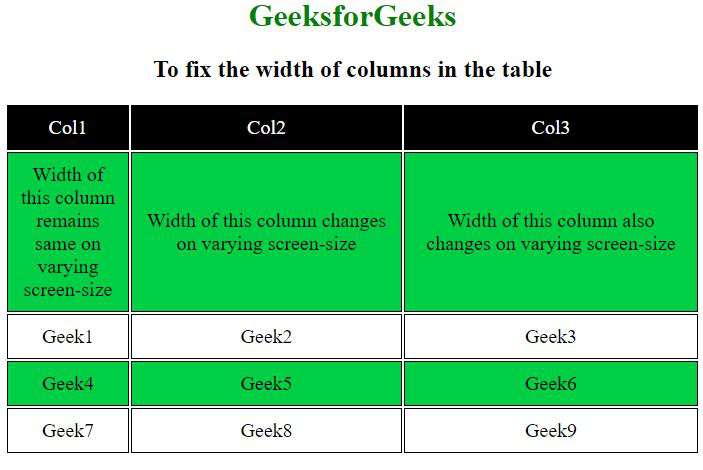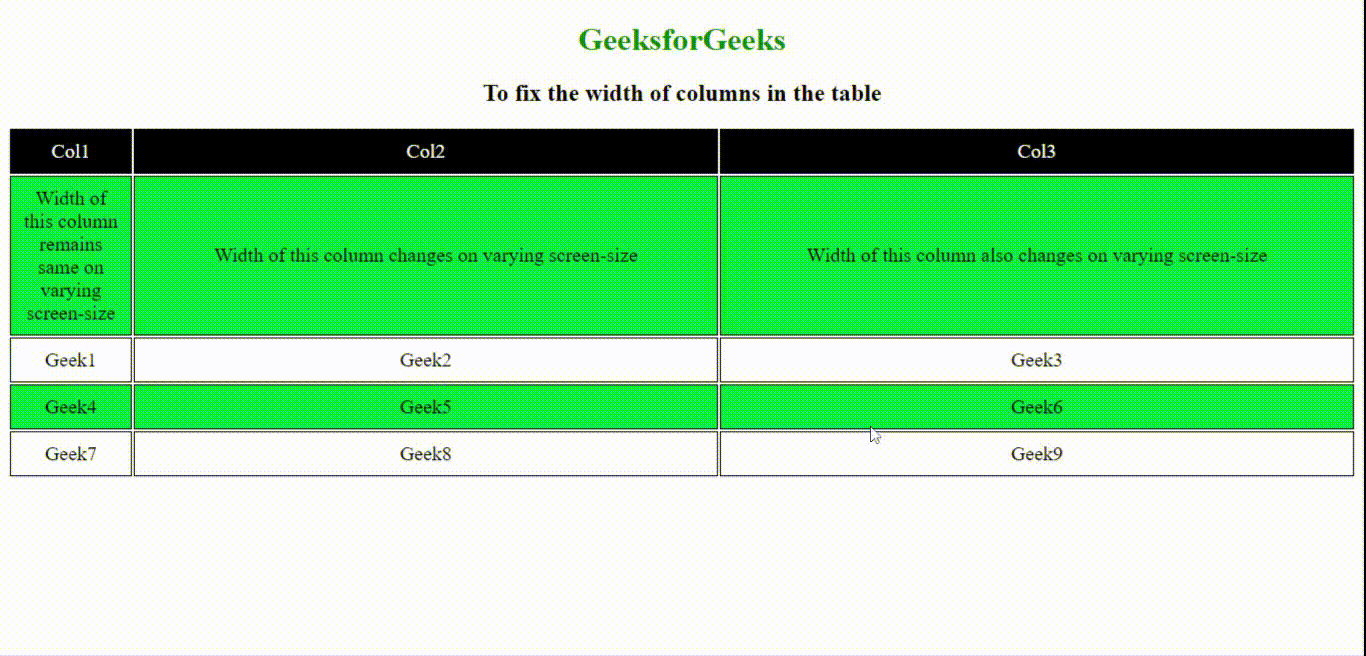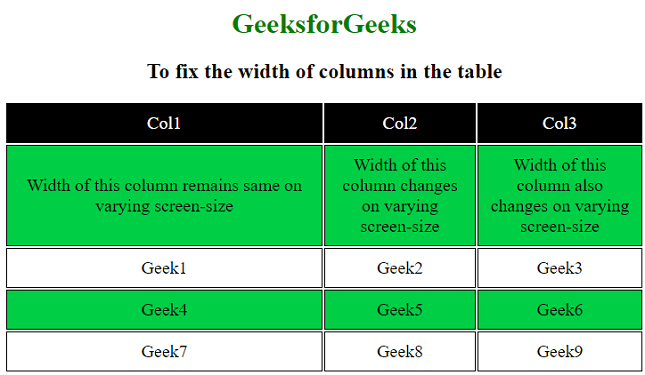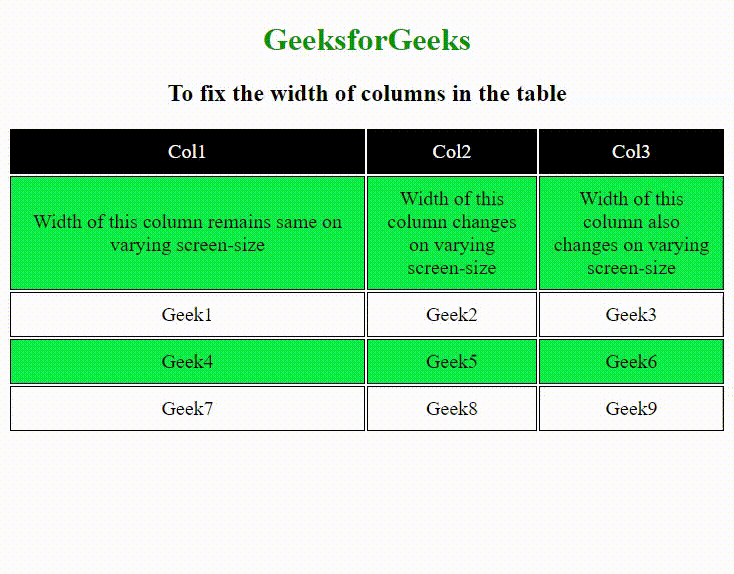# How to fix the width of columns in the table ?

We have already seen the article, How to fix the height of rows in the table.

The width of the columns i.e. td in a table can be fixed very easily. This can be done by adding the width attribute in the <td> tag. If the width is not specified, the width of the column changes according to the change in the content. The specifications of width for the columns can be in pixels, or percentage.

Syntax:

`<td width ="px | %">`

Approach:

• The width attribute is used to set the width of a column. It is added in the <td> tag.
• The width should be specified according to the content, either in pixels or percentage.
• If the content in the column is large, it will overflow.

Example 1: In this example, the width of the first column is fixed, but the width of the other columns are not fixed. So, when we change the size of the screen, the width of the first column remains the same, but the width of the other column changes according to the content. However, if the specified width is not sufficiently large according to the content, this may not work, and the first column will overflow and behave similarly to the other column.

## HTML

 ` ` `<``html``> ` ` `  `<``head``> ` `    ``<``style``> ` `        ``table { ` `            ``margin-left: auto; ` `            ``margin-right: auto; ` `            ``font-size: 20px; ` `            ``height: 100%; ` `            ``table-layout: fixed; ` `        ``} ` ` `  `        ``td { ` `            ``border: 1px solid black; ` `            ``text-align: center; ` `            ``padding: 10px; ` `        ``} ` ` `  `        ``tr:nth-child(even) { ` `            ``background-color: #00cf45; ` `        ``} ` ` `  `        ``h1 { ` `            ``color: green; ` `        ``} ` `    `` ` ` ` ` `  `<``body``> ` `    ``<``center``> ` `        ``<``h1``>GeeksforGeeks ` ` `  `        ``<``h2``> ` `            ``To fix the width of  ` `            ``columns in the table ` `        `` ` ` `  `        ``<``table``> ` `            ``<``tr` `style="color:white;  ` `                ``background-color:black;"> ` `                ``<``td` `width``=``"100px"``>Col1 ` `                ``<``td``>Col2 ` `                ``<``td``>Col3 ` `            `` ` `            ``<``tr``> ` `                ``<``td``> ` `                    ``Width of this column remains  ` `                    ``same on varying screen-size ` `                `` ` `                ``<``td``> ` `                    ``Width of this column changes  ` `                    ``on varying screen-size ` `                `` ` `                ``<``td``> ` `                    ``Width of this column also changes  ` `                    ``on varying screen-size ` `                `` ` `            `` ` `            ``<``tr``> ` `                ``<``td``>Geek1 ` `                ``<``td``>Geek2 ` `                ``<``td``>Geek3 ` `            `` ` `            ``<``tr``> ` `                ``<``td``>Geek4 ` `                ``<``td``>Geek5 ` `                ``<``td``>Geek6 ` `            `` ` `            ``<``tr``> ` `                ``<``td``>Geek7 ` `                ``<``td``>Geek8 ` `                ``<``td``>Geek9 ` `            `` ` `        `` ` `    `` ` ` ` ` `  `  `

Output: Run the following code and change the screen-size to see the difference between the columns.

• Before changing the screen-size:• After changing the screen-size:Example 2: In this example, <td width =”%”> is used.

## HTML

 ` ` `<``html``> ` ` `  `<``head``> ` `    ``<``style``> ` `        ``table { ` `            ``margin-left: auto; ` `            ``margin-right: auto; ` `            ``font-size: 20px; ` `            ``height: 100%; ` `            ``table-layout: fixed; ` `        ``} ` ` `  `        ``td { ` `            ``border: 1px solid black; ` `            ``text-align: center; ` `            ``padding: 10px; ` `        ``} ` ` `  `        ``tr:nth-child(even) { ` `            ``background-color: #00cf45; ` `        ``} ` ` `  `        ``h1 { ` `            ``color: green; ` `        ``} ` `    `` ` ` ` ` `  `<``body``> ` `    ``<``center``> ` `        ``<``h1``>GeeksforGeeks ` ` `  `        ``<``h2``> ` `            ``To fix the width of ` `            ``columns in the table ` `        `` ` ` `  `        ``<``table``> ` `            ``<``tr` `style="color:white;  ` `                ``background-color:black;"> ` `                ``<``td` `width``=``"50%"``>Col1 ` `                ``<``td``>Col2 ` `                ``<``td``>Col3 ` `            `` ` `            ``<``tr``> ` `                ``<``td``> ` `                    ``Width of this column remains  ` `                    ``same on varying screen-size ` `                `` ` `                ``<``td``> ` `                    ``Width of this column changes  ` `                    ``on varying screen-size ` `                `` ` `                ``<``td``> ` `                    ``Width of this column also changes  ` `                    ``on varying screen-size ` `                `` ` `            `` ` `            ``<``tr``> ` `                ``<``td``>Geek1 ` `                ``<``td``>Geek2 ` `                ``<``td``>Geek3 ` `            `` ` `            ``<``tr``> ` `                ``<``td``>Geek4 ` `                ``<``td``>Geek5 ` `                ``<``td``>Geek6 ` `            `` ` `            ``<``tr``> ` `                ``<``td``>Geek7 ` `                ``<``td``>Geek8 ` `                ``<``td``>Geek9 ` `            `` ` `        `` ` `    `` ` ` ` ` `  ` `

Output: The width of the column is set to “50%” , so even after changing the screen size, the width of the first column remains “50%”.

• Before changing screen-size:• After changing screen-size:My Personal Notes arrow_drop_upSmall things always make you to think big

If you like GeeksforGeeks and would like to contribute, you can also write an article using contribute.geeksforgeeks.org or mail your article to contribute@geeksforgeeks.org. See your article appearing on the GeeksforGeeks main page and help other Geeks.

Please Improve this article if you find anything incorrect by clicking on the "Improve Article" button below.